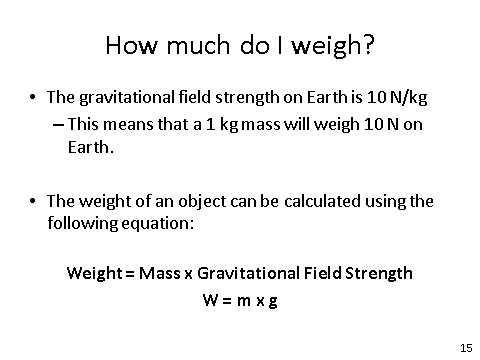# Gravity

## Weight

Weight is the force acting on an object due to gravity. The force of gravity close to the Earth is due to the gravitational field around the Earth. The weight of an object is always considered to be acting at a single point referred to as the ‘centre of mass’/ The weight of an object is directly proportional to the mass. Directly proportional: means that there is a linear relationship (straight line) and goes through the origin (of a graph). Learn this definition, it can be worth up to two marks!! If ever you write it in an exam - define it straight away.

## Calculating Weight

The equation for weight is one that you need to be able to recall and apply.Weight is measured in newtons (N).

Mass is measured in kilograms (kg).

Gravitational Field Strength is measured in newtons per kilograms (N/kg).

In any calculation, you will always be given a value for g.

## Measuring Weight

To measure weight, you can use something called a newtonmeter. As shown in the picture below, you hang your item on the hook and then read off the scale. There are different newtonmeters for different weights, but they all work the same.Calculate the weight for an object which has a mass of 56kg. g = 9.8N/kg